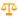# Scale

 Block Group: Math Operations Icon:The Scale block returns the result of re-scaling a number from one defined set of lower and upper limits to another.

Evaluation of the result is according to the following formula:

`(input – inputMin) * ((outputMax – outputMin) / (inputMax – inputMin)) = output`

For information on using dataflow blocks, see Dataflow.

For accepted and excluded arguments of Math Operations blocks, see Math Operations.

## Input/Output Properties

The following properties of the Scale block can take input and give output.

• input (number)
• inputMin (number)
• inputMax (number)
• outputMin (number)
• outputMax (number)
• inputOutOfRange (boolean)

input specifies the number to re-scale.

inputMin specifies the minimum of the original scale.

inputMax specifies the maximum of the original scale.

outputMin specifies the minimum of the new scale.

outputMax specifies the maximum of the new scale.

inputOutOfRange returns TRUE if the input is outside the range, FALSE otherwise.

## Output Property

The following property of the Scale block can give output but cannot take input.

• output (number)

output returns the value in the new scale that corresponds to input in the old scale. If input is below the input range, output returns outputMin. If input is above the input range, output returns outputMax.

## Examples

The following image shows four examples of the Scale block.